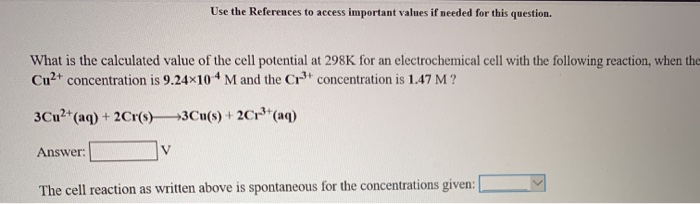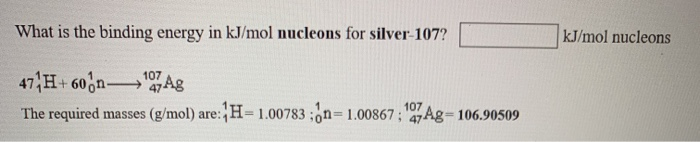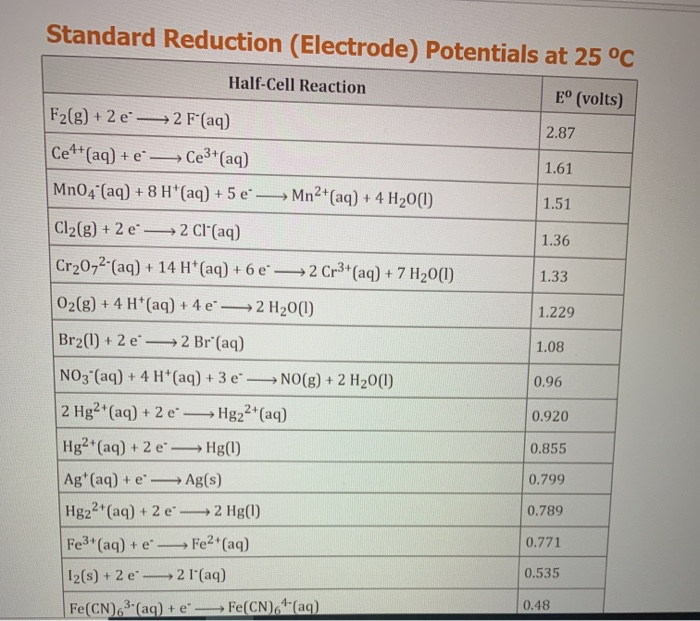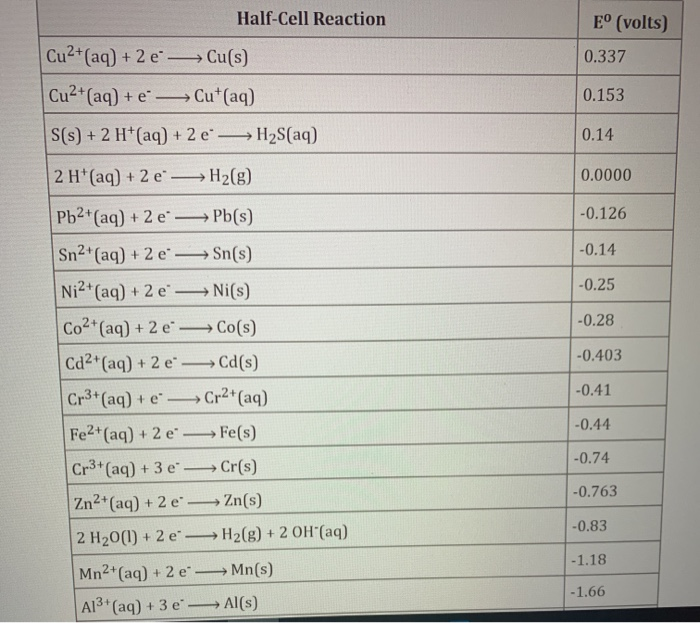# Help with these please Use the References to access important values if needed for this question....

###### Question:Use the References to access important values if needed for this question. What is the calculated value of the cell potential at 298K for an electrochemical cell with the following reaction, when the Cu2 concentration is 9.24x104 M and the Cr3 concentration is 1.47 M ? 3Cu2 (aq)+2Cr(s)3Cu(s) + 2Cr (aq) V Answer: The cell reaction as written above is spontaneous for the concentrations given:
What is the binding energy in kJ/mol nucleons for silver-107? kJ/mol nucleons 47 H+ 60n7Ag 107 107 The required masses (g/mol) are:H-1.007830n- 1.00867;47Ag 106.90509
Standard Reduction (Electrode) Potentials at 25 °C Half-Cell Reaction E (volts) F2(g)+2 e 2 F (aq) 2.87 Ce4 (aq) + e Ce3 (aq) 1.61 MnO4 (aq) +8 H*(aq) +5 e Mn2 (aq) + 4 H20(I) 1.51 Cl2(g) +2 e 2 Cl'(aq) 1.36 Cr2072 (aq) + 14 H (aq) + 6 e 2 Cr3 (aq)+ 7 H20(1) 1.33 02(g) + 4 H*(aq) + 4 e" 2 H20(1) 1.229 Br2(1)+2 e 2 Br (aq) 1.08 NO3 (aq)+ 4 H*(aq)+ 3 e- NO(g)+ 2 H20() 0.96 2 Hg2 (aq)+ 2 e Hg22 (aq) 0.920 Hg2 (aq) + 2 e Hg() 0.855 Ag (aq) + e Ag(s) 0.799 Hg22 (aq) + 2 e 2 Hg(l) 0.789 Fe3 (aq)+ e Fe2 (aq) 0.771 2 I'(aq) 12(s) +2 e 0.535 Fe(CN)4 (aq) Fe(CN)3-(aq) + e 0.48
Half-Cell Reaction Eo (volts) Cu2 (aq) + 2 e Cu(s) 0.337 Cu2 (aq) + e Cu (aq) 0.153 S(s)+2 H*(aq) +2 e' H2S(aq) 0.14 2 H'(aq)+ 2 e-H2(g) 0.0000 Pb2 (aq)+ 2 e -0.126 Pb(s) Sn2 (aq) +2 e -0.14 Sn(s) -0.25 Ni2 (aq) + 2 e-Ni(s) -0.28 Co2 (aq) + 2 e-Co(s) -0.403 Cd2 (aq)+ 2 e Cd(s) -0.41 Cr3 (aq)+ e Cr2 (aq) -0.44 Fe2 (aq) + 2 e Fe(s) -0.74 Cr3 (aq) + 3 e- Cr(s) -0.763 Zn2 (aq) + 2 e- Zn(s) -0.83 H2(g) + 2 OH'(aq) 2 H20(1)+ 2 e -1.18 Mn2 (aq) + 2 e Mn(s) -1.66 A13 (aq) + 3 e-Al(s)

#### Similar Solved Questions

##### Complete each sentence with 1) equal 4) reverse 2) not equal 3) forward 5) changes 6)...
Complete each sentence with 1) equal 4) reverse 2) not equal 3) forward 5) changes 6) does not change A. Reactants form products in the reaction. B. At equilibrium, the reactant concentration C. Products form reactants in the reaction....
##### In a population where there is a heterozygous advantage, if the relative fitness of the A1A1(W11)...
In a population where there is a heterozygous advantage, if the relative fitness of the A1A1(W11) genotype is 0.6, A1A2/W12) is 1.0, and A2A2(W22) is 0.9, at equilibrium the frequency of the A2 allele will be: 0 0.2 . 0.6 0.8 0.4...
##### What type of gradient is critical to ATP formation by oxidative phosphorylation? A. sodium ion B....
What type of gradient is critical to ATP formation by oxidative phosphorylation? A. sodium ion B. chloride ion C. proton D. potassium ion E. None of the answers is correct. Which of the following citric acid cycle intermediates would become depleted if malonate, a compet...
##### Using activity-based costing to make decisions Treat Dog Collars uses activity-based costing. Treat's system has the...
Using activity-based costing to make decisions Treat Dog Collars uses activity-based costing. Treat's system has the following features Predetermined Overhead Allocation Rate Activity Allocation Base Purchasing Number of pu chase orders 60.00 per purchase order Assembling Packaging Number of par...